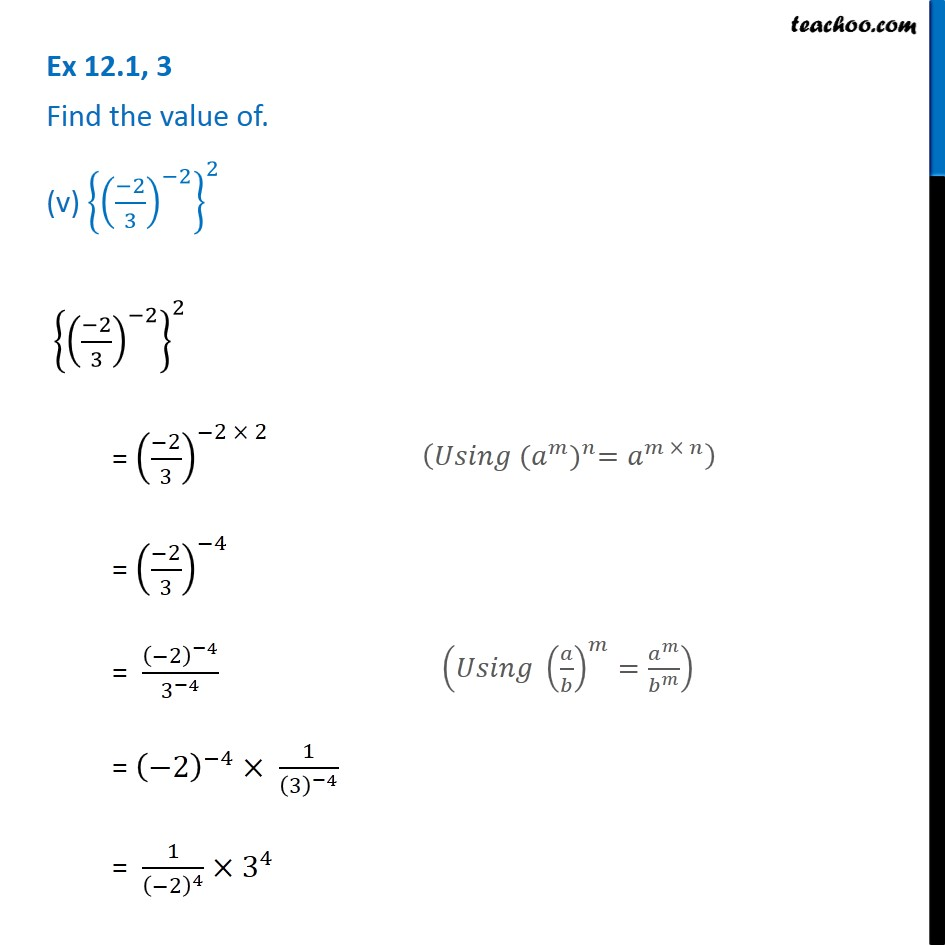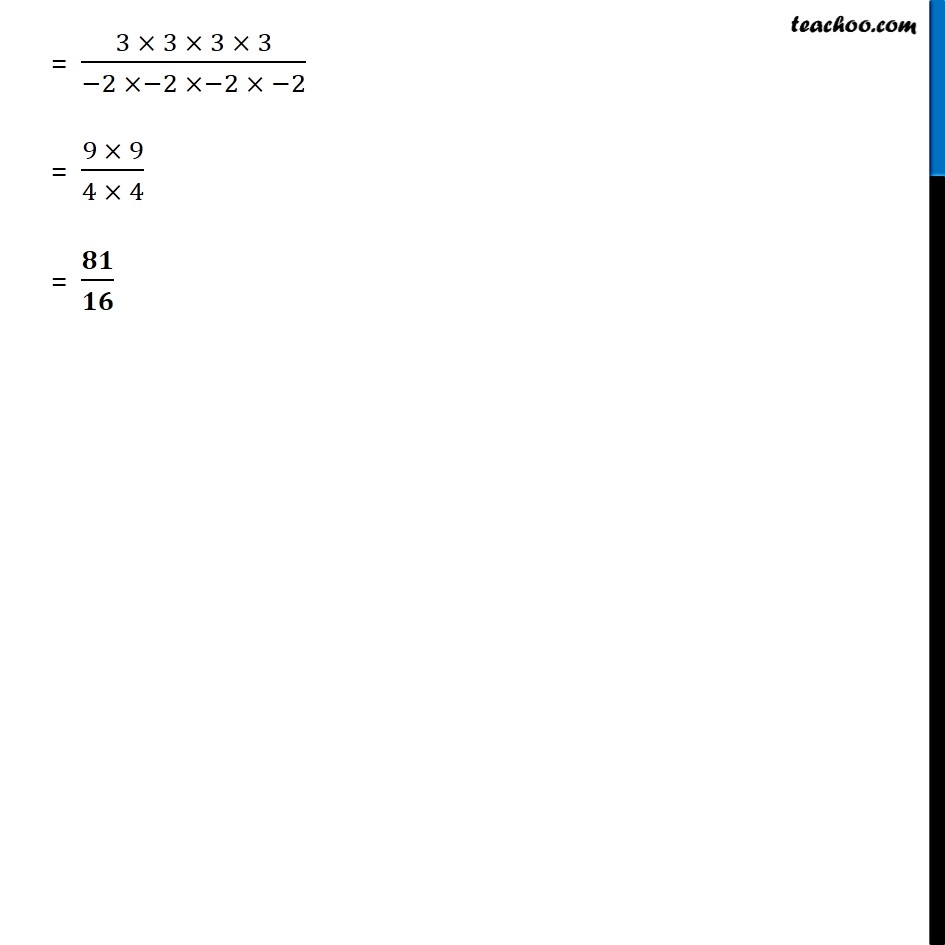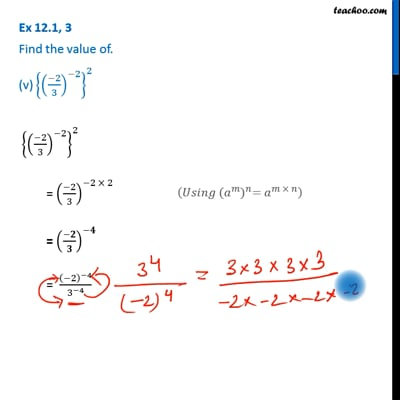Ex 12.1

Chapter 12 Class 8 Exponents and Powers
Serial order wiseThis video is only available for Teachoo black users

Get live Maths 1-on-1 Classs - Class 6 to 12

### Transcript

Ex 12.1, 3 Find the value of. (v) {((−2)/3)^(−2) }^2 {((−2)/3)^(−2) }^2 = ((−2)/3)^(−2 × 2) = ((−2)/3)^(−4) = (−2)^(−4)/3^(−4) = (−2)^(−4)× 1/(3)^(−4) = 1/(−2)^4 ×3^4 (𝑈𝑠𝑖𝑛𝑔 〖(𝑎^𝑚)〗^𝑛=𝑎^(𝑚 × 𝑛) ) (𝑈𝑠𝑖𝑛𝑔 (𝑎/𝑏)^𝑚=𝑎^𝑚/𝑏^𝑚 ) = (3 × 3 × 3 × 3)/(−2 ×−2 ×−2 × −2) = (9 × 9)/(4 × 4) = 𝟖𝟏/𝟏𝟔